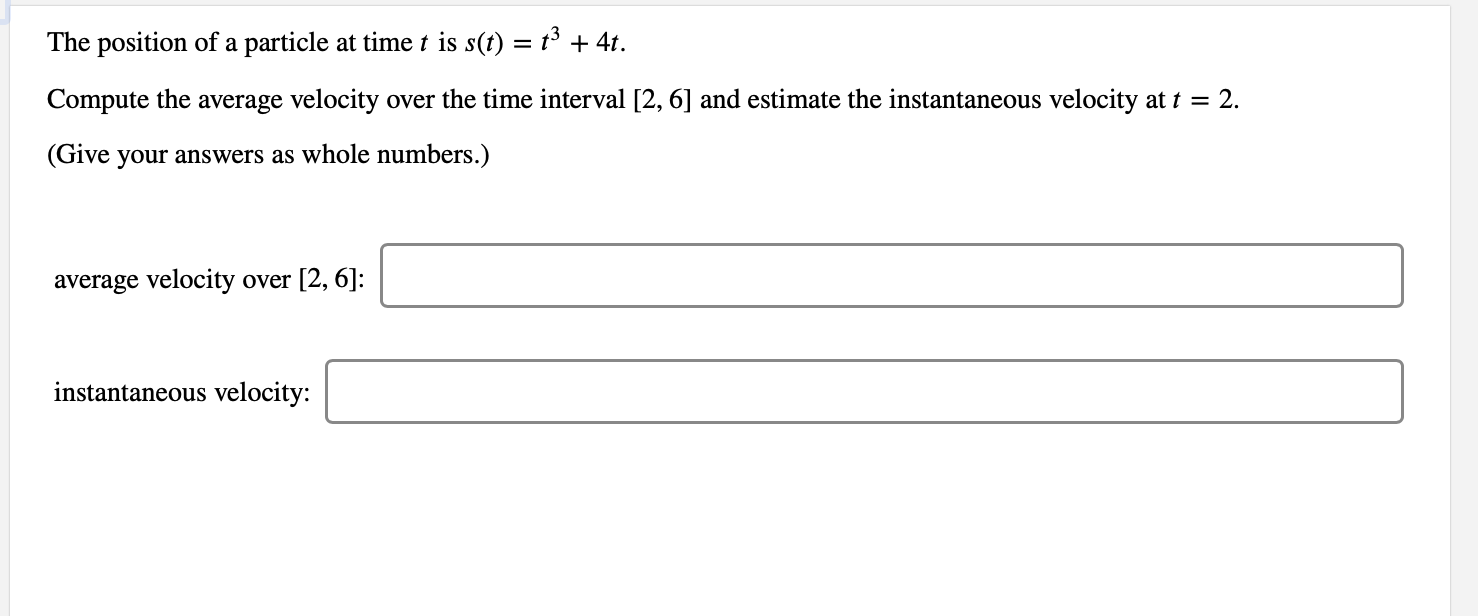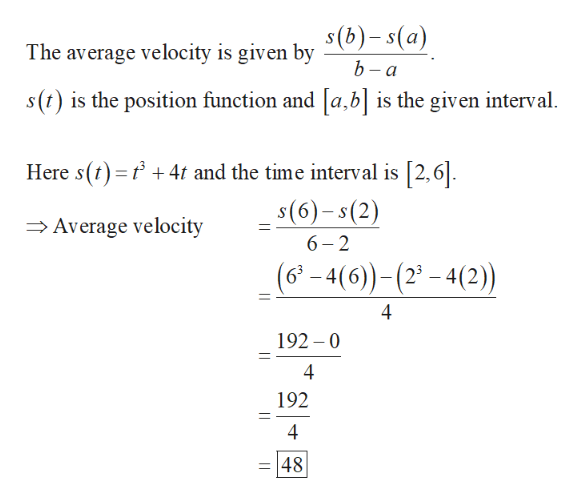# The position of a particle at time t is s(t) = t3 + 4tover the time interval [2, 6] and estimate the instantaneous velocity at t = 2Compute the average velocity(Give your answers as whole numbers.)average velocity over [2, 6]:instantaneous velocity:

Question
3 viewshelp_outlineImage TranscriptioncloseThe position of a particle at time t is s(t) = t3 + 4t over the time interval [2, 6] and estimate the instantaneous velocity at t = 2 Compute the average velocity (Give your answers as whole numbers.) average velocity over [2, 6]: instantaneous velocity: fullscreen
check_circle

Step 1

Obtain the average velocity of the given parti...help_outlineImage TranscriptioncloseThe average velocity is given bv S(b)- s(a) b-a s(t) is the position function and [a, b] is the given interval 4 and the time interval is [2,6 Here s() s(6)-s(2) 6 2 Average velocity (6-4(6)-(-4(2) 4 192 0 4 192 4 48 fullscreen

### Want to see the full answer?

See Solution

#### Want to see this answer and more?

Solutions are written by subject experts who are available 24/7. Questions are typically answered within 1 hour.*

See Solution
*Response times may vary by subject and question.
Tagged in

### Derivative